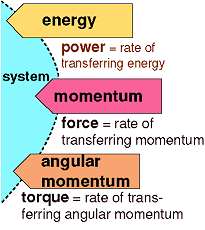Lectures on Physics has been derived from Benjamin Crowell's Light and Matter series of free introductory textbooks on physics. See the editorial for more information....# Torque: the Rate of Transfer of Angular Momentum

Force can be interpreted as the rate of transfer of momentum. The equivalent in the case of angular momentum is called torque(rhymes with fork). Where force tells us how hard we are pushing or pulling on something, torque indicates how hard we are twisting on it. Torque is represented by the Greek letter tau, τ , and the rate of change of an object's angular momentum equals the total torque acting on it,(If the angular momentum does not change at a constant rate, the total torque equals the slope of the tangent line on a graph of L versus t.)Energy, momentum, and angular momentum can be transferred. The rates of transfer are called power, force, and torque.

As with force and momentum, it often happens that angular momentum recedes into the background and we focus our interest on the torques. The torque-focused point of view is exemplified by the fact that many scientifically untrained but mechanically apt people know all about torque, but none of them have heard of angular momentum. Car enthusiasts eagerly compare engines' torques, and there is a tool called a torque wrench which allows one to apply a desired amount of torque to a screw and avoid overtightening it.

Last Update: 2010-11-11